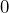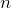# P. Sousi: The Uniform Spanning Tree in 4 dimensions

Abstract: A uniform spanning tree ofcan be thought of as the ‘‘uniform measure’’ on trees of. The past ofin the uniform spanning tree is the finite component that is disconnected from infinity whenis deleted from the tree. We establish the logarithmic corrections to the probabilities that the past contains a path of length, that it has volume at leastand that it reaches the boundary of the box of side lengtharound. Dimension 4 is the upper critical dimension for this model in the sense that in higher dimensions it exhibits “mean-field” critical behaviour. An important part of our proof is the study of the Newtonian capacity of a loop erased random walk in 4 dimensions. This is joint work with Tom Hutchcroft.

## Video